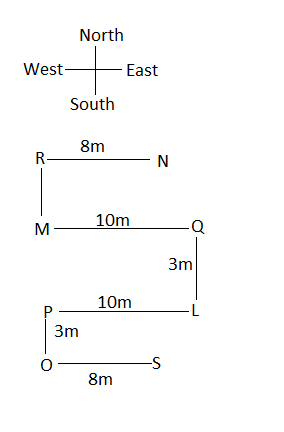# Reasoning Ability Quiz: 8 March 2021

Updated Mon, 08 Mar 2021 03:28 PM IST

### Free Demo Classes

Q: 1-4)
Direction: Study the information carefully and answer the question that follows.

Eight persons L, M, N, O, P, Q, R, and S. R is 3m towards the north of M and is 8m towards the west of N.L is 3m towards the south of Q. Q is 10m towards east of M. P is 10m towards the west of L and is 3m towards the north of O. O is 8m towards the west of S.

Q-1) What is the direction of R with respect to O?
A)South
B)North
C)South-west
D)North-east
E)None of these

Q-2) What is the shortest distance between N and S ?
A)9m
B)10m
c)3m
D) can not be determine
E) None of these

Q-3) Four of the following five are alike in a certain way and so form a group.Source: safalta.com

Which is the one that does not belong to the group?
A) RN
B) OS
C) MQ
D) PL
E) MP

Q-4) If the distance between point L and A is 2m then what is the shortest distance between R and A?
A)10m
B)12m
c)15m
D)8m
E) can not be determine

Q: 5-8)
Direction : The following question are based on the five three digit number , Solve the following question based on this three digit number.

621    297    333     786    377
Q- 5) If first and the last digit within the number is interchange and then arranged in increasing order then which of the following is the highest number?
1. 621
2. 297
3. 333
4. 786
5. 377

Q-6) 621    297    333     786    377
If one is subtracted from the second digit of each number and one is added to the last digit digit of each number then Which number is not divisible by 2?
1. 621
2. 297
3. 333
4. 786
5. 377
Q-7)  621    297    333     786    377
If in each number three digit are arranged in increasing order, which of the following is the second lowest number?
1. 621
2. 297
3. 333
4. 786
5. 377
Q-8) 621    297    333     786    377
If second digit of the second number is divided by the first digit of last number than what is the resultant?
1. 2
2. 3
3. 4
4. 5
5. None of these
Q-9) if in the number 748538798, 1 is added to each of the odd digit which is more than 5 and after that  subtract 1 from each digit which is less than or equal to 5. Then how many numbers are repeating in the formed number?
1. None
2. One
3. Two
4. Three
5. More than three
Q-10) Q-9) if in the number 748538798, 1 is added to each of the odd digit which is more than 5 and after that  subtract 1 from each digit which is less than or equal to 5. Then which number is 5th to the left end of the number thus formed?

1. 9
2. 8
3. 2
4. 4
5. 3

Solution: -
Q: 1-4)ANS-1)  B)North
ANS-2)  A) 9m
ANS-3)  E) MP
Ans – E) can not be determine
Because it is not clear that A is in Which direction with respect to L

Q:5-8)

ANS-5) 297

SOLUTION -
Given :- 621    297    333     786    377
On change of first and the last digit within the number
126      792     333     687     773
On arranged in increasing order, We get
126     333      687       773     792
Hence the highest number is  - 792  or 297

ANS-6) 786
SOLUTION:
SOLUTION -
Given :- 621    297    333     786    377

ANS-7)–  297

Solution:
Given :- 621    297    333     786    377
On arranged in increasing order, We get
126     279       333       678      377
Hence second lowest number is 297

ANS-8) Ans – 3

Solution
second digit of the second number = 9
and, first digit of last number = 3
Hence the resultant is 3

Ans-9)

Two

Solution
Given number = 748538798
Odd digit – 7, 8, 3, 7, 8
1 is added to each of the odd digit which is more than 5
= 7+1, 8+1, 7+1, 8+1
=8,9,8,9
Thus the number formed = 849538899
Now,
subtract 1 from each digit which is less than or equal to 5 = 4, 5, 3
After subtracting 1, we get
3, 4, 2
Hence the formed number = 839428899
Repeating number are – 8, 9

ANS –10)  C) 2

Given number = 748538798
Odd digit – 7, 8, 3, 7, 8
1 is added to each of the odd digit which is more than 5
= 7+1, 8+1, 7+1, 8+1
=8,9,8,9
Thus the number formed = 849538899
Now,
subtract 1 from each digit which is less than or equal to 5 = 4, 5, 3
After subtracting 1, we get
3, 4, 2
Hence the formed number = 839428899
5th to the left end of the number thus formed = 2

## Start Learning & Earning

### Trending Courses##### Master Certification in Digital Marketing Programme (Batch-8)

Now at just ₹ 64999 ₹ 12500048% off##### Professional Certification Programme in Digital Marketing (Batch-2)

Now at just ₹ 49999 ₹ 12500060% off##### Advanced Certification in Digital Marketing Online Programme (Batch-19)

Now at just ₹ 24999 ₹ 4999950% off##### Advance Certification In Graphic Design Programme (Batch-8) : 100 Hours Live Interactive Classes

Now at just ₹ 15999 ₹ 2999947% off##### Advanced Certification in Digital Marketing Classroom Programme (Batch-3)

Now at just ₹ 29999 ₹ 9999970% offNow at just ₹ 599 ₹ 159963% off